# NCERT Solutions for Chapter 2 Shapes And Angles Class 5 Maths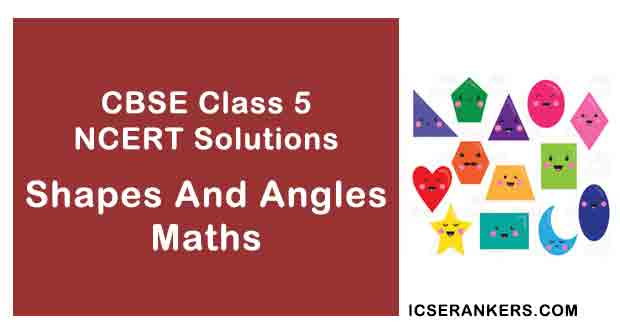Shapes And Angles Questions and Answers

 Chapter Name Shapes And Angles NCERT Solutions Class CBSE Class 5 Textbook Name Maths Related Readings NCERT Solutions for Class 5NCERT Solutions for Class 5 Maths Summary of Shapes And Angles

Page No 18:

Question 1:

Look at the shape and answer.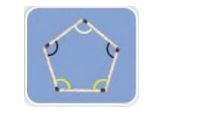• The angle marked in _______ colour is the biggest angle.

All the angles in the figure are equal.

Question 2:

(a) Are the angles marked with yellow equal?

(b) Are the angles marked with green equal?

(c) Are the angles marked with blue equal?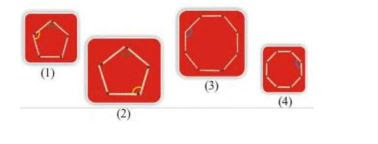(a) The angles marked with yellow are equal.

(b) The angles marked with green are equal.

(c) The angles marked with blue are equal.

Question 3: Four different angles are marked in four colours. Can you find other angles which are the same as the one marked in red? Mark them in red. Do this for the other colours.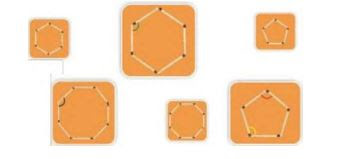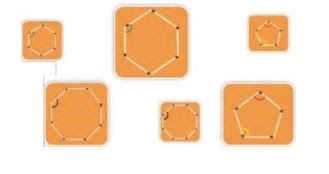Page No 19:

Question 1: How many different shapes can you make by changing the

angle between the matchsticks in each of these? Try.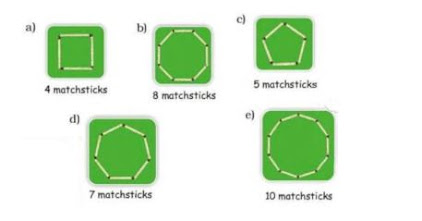Page No 21:

Question 1: Go around with your tester and draw here those things in which the tester opens like the letter L. Are you sure they are all right angles?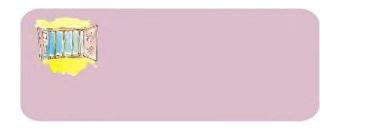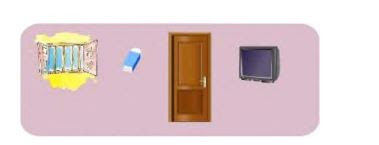Disclaimer: The purpose of this section is to make students observe their surroundings. The answer may vary from student to student. It is highly recommended that the students prepare the answer on their own.

Question 2: Look at the angles in the pictures and fill the table.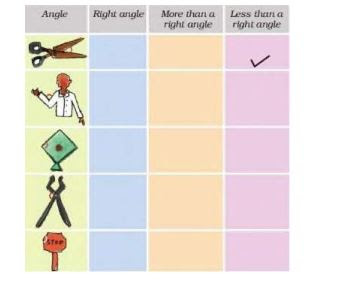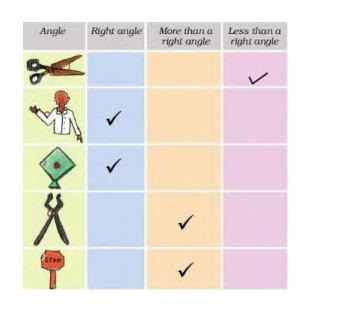Page No 22:

Question 1: Sukhman made this picture with so many angles.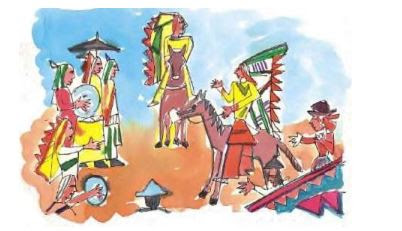Use colour pencils to mark.

• right angles with black colour.
• angles which are more than a right angle with green.
• angles which are less than a right angle with blue.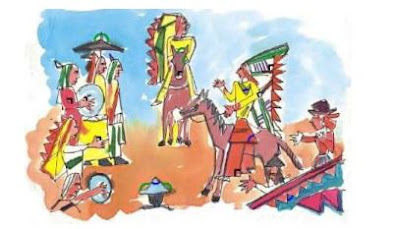Question 2: Draw anything of your choice around the angle shown. Also write what kind of angle it is. The first one is done.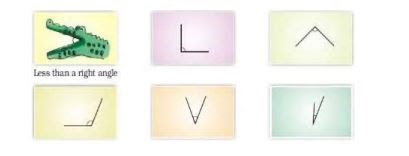Disclaimer: The purpose of this section is to make students observe their surroundings. It is highly recommended that the students prepare the answers on their own.

Page No 25:

Question 1: Write 3 names using straight lines and count the angles.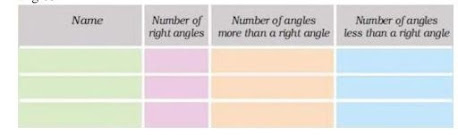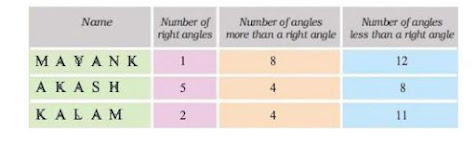Page No 26:

Question 1: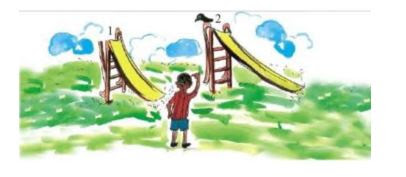These are two slides in a park.

• Which slide has a larger angle
• Which slide do you think is safer for the little boy? Why?

Slide I has a larger angle.
• As slide 1 has a larger angle and steeper than slide 2, there will be more chances for the boy to get hurt while sliding on slide 1. Thus, slide 2 would be safer for the little boy than slide 1.

Page No 28:

Question 1: Shapes and Towers Look for triangles in the pictures below.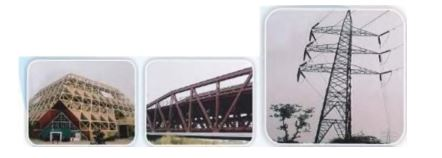• From the activity 'Changing Shapes' can you guess why triangles are used in these towers, bridges   etc.?
• Look around and find out more places where triangles are used.

• The triangles are used in the towers and bridges so as to make them strong. We know that a triangle is the strongest polygon out of all the other polygons. The angles can get changed in other polygons. However, in a triangle, the angles cannot change once the triangle is built
Disclaimer: The purpose of this section is to make the students observe their surroundings. The answer may vary from student to student based on his/her experience. It is highly recommended that the students prepare the answers on their own. The answer provided here is for reference only.

Question 2: There are many times in a day when the hands of a clock make a right angle. Now you draw some more.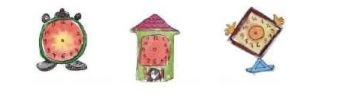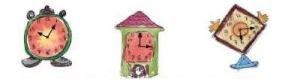Disclaimer: The answer may vary from student to student, based on his/her observation. It is highly recommended that the students prepare the answer on their own. The answer provided here is for reference only.

Page No 29:

Question 1: Write what kind of angle is made by the hands at these times. Also write the time.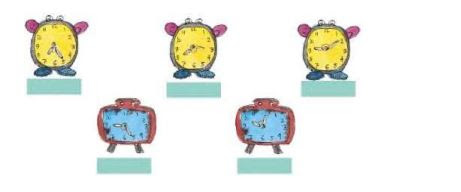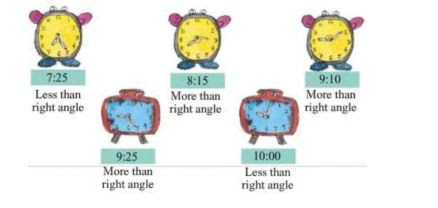Question 2: Draw the hands of the clock when they make an angle which is less than a right angle. Also write the time.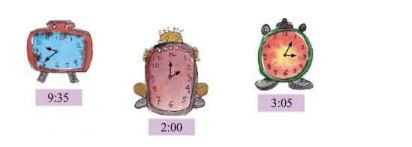Disclaimer: The answer may vary from student to student, based on his/her observation. It is highly recommended that the students prepare the answer on their own.

Page No 31:

Question 1: Can you guess how many degrees is the angle which is

• 12 of a right angle __________
• 13 of a right angle __________
•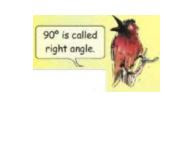2 times of a right angle __________